# Specific Heats of Gases

The specific heats of gases are generally expressed as molar specific heats. For a monoatomic ideal gas the internal energy is all in the form of kinetic energy, and kinetic theory provides the expression for that energy, related to the kinetic temperature. The expression for the internal energy isTwo specific heats are defined for gases, one for constant volume (CV) and one for constant pressure (CP). For a constant volume process with a monoatomic ideal gas the first law of thermodynamics gives: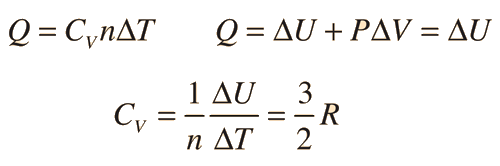More general case

Further application of the ideal gas law and first law gives the relationshipShow

The ratio of the specific heats γ = CP/CV is a factor in adiabatic engine processes and in determining the speed of sound in a gas. This ratio γ = 1.66 for an ideal monoatomic gas and γ = 1.4 for air, which is predominantly a diatomic gas.

Index

Gas law concepts

Kinetic theory concepts

 HyperPhysics***** Thermodynamics R Nave
Go Back

# Constant Volume Specific Heat

The molar specific heat at constant volume is defined byConstant volume

Using the first law of thermodynamics this can be put in the formbut since ΔV = 0, the expression for CV becomes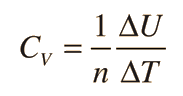For a monoatomic ideal gas,, soThis value agrees well with experiment for monoatomic noble gases such as helium and argon, but does not describe diatomic or polyatomic gases since their molecular rotations and vibrations contribute to the specific heat. The equipartition of energy predicts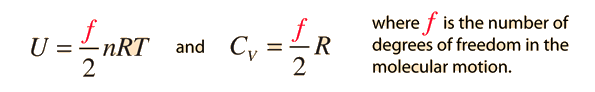Index

Gas law concepts

Kinetic theory concepts

 HyperPhysics***** Thermodynamics R Nave
Go Back

# Constant Pressure Specific Heat

The molar specific heat at constant pressure is defined byConstant pressure
Using the first law of thermodynamics for a constant pressure process this can be put in the formFrom the ideal gas law (PV=nRT) under constant pressure conditions it can be seen thatSince the constant volume specific heat isit follows thatFor an ideal monoatomic gasIndex

Kinetic theory concepts

 HyperPhysics***** Thermodynamics R Nave
Go Back

# Molar Specific Heats of Gases

The molar specific heats of ideal monoatomic gases are: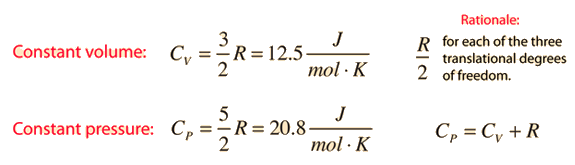For diatomic or linear polyatomic molecules, two rotational degrees of freedom are added, corresponding to the rotation about two perpendicular axes through the center of the molecule. This would be expected to give CV = 5/2 R, which is borne out in examples like nitrogen and oxygen. A nonlinear polyatomic molecule will be able to rotate about three perpendicular axes, which would be expected to give CV = 3R. The departure from this value which is observed indicates that vibrational degrees of freedom must also be included for a complete description of specific heats of gases.

 Examples Table of molar heat capacities
Index

Kinetic theory concepts

Sears & Salinger, Sec 9-7

 HyperPhysics***** Thermodynamics R Nave
Go Back

# Selected Specific HeatsThe models of constant-volume specific heat based on equipartition of energy and including rotational degrees of freedom as well as translational are able to explain specific heats for diatomic molecules. The departure from this model in the case of non-linear polyatomic molecules indicates vibrational involvement.

The constant pressure specific heat is related to the constant volume value by CP = CV + R. The ratio of the specific heats γ = CP/CV is a factor in adiabatic engine processes and in determining the speed of sound in a gas.

### Hydrogen as example of diatomic molecule

Index

Kinetic theory concepts

Sears & Salinger, Sec 9-7

 HyperPhysics***** Thermodynamics R Nave
Go Back

# Hydrogen Specific Heat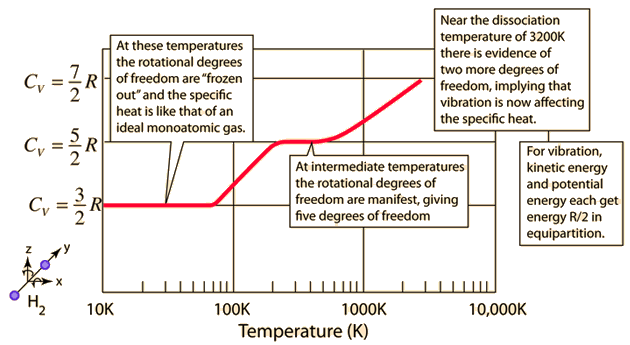The behavior of the specific heat of hydrogen with changing temperature was extremely puzzling early in the 20th century. At low temperatures it behaved like a monoatomic gas, but at higher temperatures its specific heat took on a value similar to other diatomic molecules. It took the development of the quantum theory to show that diatomic hydrogen, with its tiny rotational inertia, required a large amount of energy to excite its first excited molecular rotation quantum state. Since it could not get that amount of energy at low temperatures, it acted like a monoatomic gas.

 Specific heats of gases Equipartition of energy
Index

Kinetic theory concepts

 HyperPhysics***** Thermodynamics R Nave
Go Back